This notebook was put together by [Jake Vanderplas](http://www.vanderplas.com) for PyCon 2015. Source and license info is on [GitHub](https://github.com/jakevdp/sklearn_pycon2015/).

# Supervised Learning In-Depth: Support Vector Machines¶

Previously we introduced supervised machine learning. There are many supervised learning algorithms available; here we'll go into brief detail one of the most powerful and interesting methods: Support Vector Machines (SVMs).

In :
%matplotlib inline
import numpy as np
import matplotlib.pyplot as plt
from scipy import stats

# use seaborn plotting defaults
import seaborn as sns; sns.set()


## Motivating Support Vector Machines¶

Support Vector Machines (SVMs) are a powerful supervised learning algorithm used for classification or for regression. SVMs are a discriminative classifier: that is, they draw a boundary between clusters of data.

Let's show a quick example of support vector classification. First we need to create a dataset:

In :
from sklearn.datasets.samples_generator import make_blobs
X, y = make_blobs(n_samples=50, centers=2,
random_state=0, cluster_std=0.60)
plt.scatter(X[:, 0], X[:, 1], c=y, s=50, cmap='spring');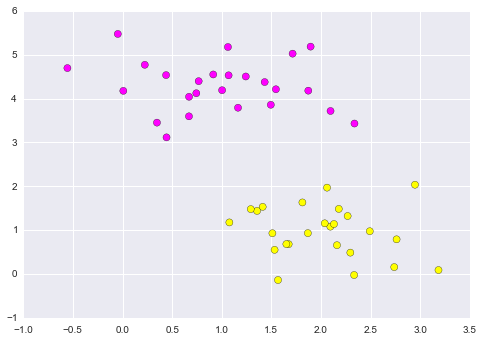A discriminative classifier attempts to draw a line between the two sets of data. Immediately we see a problem: such a line is ill-posed! For example, we could come up with several possibilities which perfectly discriminate between the classes in this example:

In :
xfit = np.linspace(-1, 3.5)
plt.scatter(X[:, 0], X[:, 1], c=y, s=50, cmap='spring')

for m, b in [(1, 0.65), (0.5, 1.6), (-0.2, 2.9)]:
plt.plot(xfit, m * xfit + b, '-k')

plt.xlim(-1, 3.5);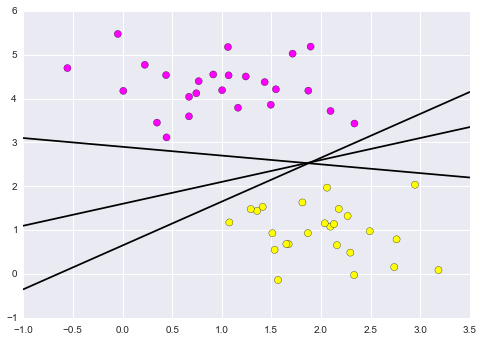These are three very different separaters which perfectly discriminate between these samples. Depending on which you choose, a new data point will be classified almost entirely differently!

How can we improve on this?

### Support Vector Machines: Maximizing the Margin¶

Support vector machines are one way to address this. What support vector machined do is to not only draw a line, but consider a region about the line of some given width. Here's an example of what it might look like:

In :
xfit = np.linspace(-1, 3.5)
plt.scatter(X[:, 0], X[:, 1], c=y, s=50, cmap='spring')

for m, b, d in [(1, 0.65, 0.33), (0.5, 1.6, 0.55), (-0.2, 2.9, 0.2)]:
yfit = m * xfit + b
plt.plot(xfit, yfit, '-k')
plt.fill_between(xfit, yfit - d, yfit + d, edgecolor='none', color='#AAAAAA', alpha=0.4)

plt.xlim(-1, 3.5);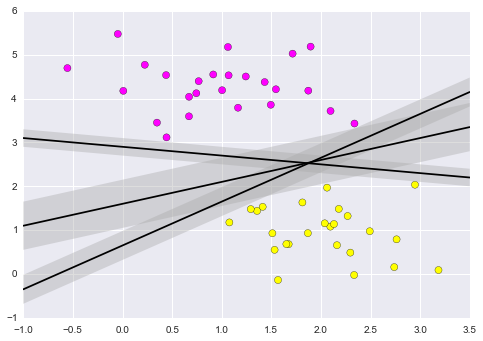Notice here that if we want to maximize this width, the middle fit is clearly the best. This is the intuition of support vector machines, which optimize a linear discriminant model in conjunction with a margin representing the perpendicular distance between the datasets.

#### Fitting a Support Vector Machine¶

Now we'll fit a Support Vector Machine Classifier to these points. While the mathematical details of the likelihood model are interesting, we'll let you read about those elsewhere. Instead, we'll just treat the scikit-learn algorithm as a black box which accomplishes the above task.

In :
from sklearn.svm import SVC # "Support Vector Classifier"
clf = SVC(kernel='linear')
clf.fit(X, y)

Out:
SVC(C=1.0, cache_size=200, class_weight=None, coef0=0.0, degree=3, gamma=0.0,
kernel='linear', max_iter=-1, probability=False, random_state=None,
shrinking=True, tol=0.001, verbose=False)

To better visualize what's happening here, let's create a quick convenience function that will plot SVM decision boundaries for us:

In :
def plot_svc_decision_function(clf, ax=None):
"""Plot the decision function for a 2D SVC"""
if ax is None:
ax = plt.gca()
x = np.linspace(plt.xlim(), plt.xlim(), 30)
y = np.linspace(plt.ylim(), plt.ylim(), 30)
Y, X = np.meshgrid(y, x)
P = np.zeros_like(X)
for i, xi in enumerate(x):
for j, yj in enumerate(y):
P[i, j] = clf.decision_function([xi, yj])
# plot the margins
ax.contour(X, Y, P, colors='k',
levels=[-1, 0, 1], alpha=0.5,
linestyles=['--', '-', '--'])

In :
plt.scatter(X[:, 0], X[:, 1], c=y, s=50, cmap='spring')
plot_svc_decision_function(clf);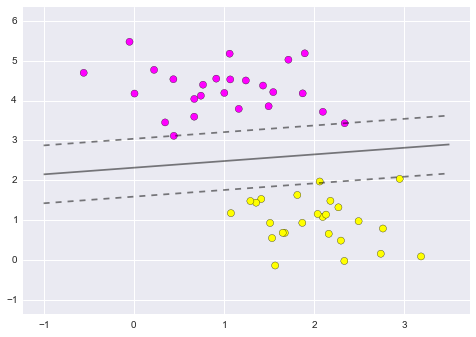Notice that the dashed lines touch a couple of the points: these points are the pivotal pieces of this fit, and are known as the support vectors (giving the algorithm its name). In scikit-learn, these are stored in the support_vectors_ attribute of the classifier:

In :
plt.scatter(X[:, 0], X[:, 1], c=y, s=50, cmap='spring')
plot_svc_decision_function(clf)
plt.scatter(clf.support_vectors_[:, 0], clf.support_vectors_[:, 1],
s=200, facecolors='none');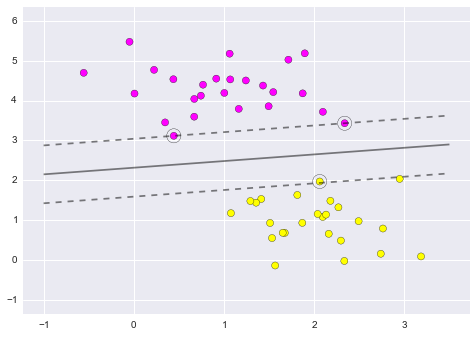Let's use IPython's interact functionality to explore how the distribution of points affects the support vectors and the discriminative fit. (This is only available in IPython 2.0+, and will not work in a static view)

In :
from IPython.html.widgets import interact

def plot_svm(N=10):
X, y = make_blobs(n_samples=200, centers=2,
random_state=0, cluster_std=0.60)
X = X[:N]
y = y[:N]
clf = SVC(kernel='linear')
clf.fit(X, y)
plt.scatter(X[:, 0], X[:, 1], c=y, s=50, cmap='spring')
plt.xlim(-1, 4)
plt.ylim(-1, 6)
plot_svc_decision_function(clf, plt.gca())
plt.scatter(clf.support_vectors_[:, 0], clf.support_vectors_[:, 1],
s=200, facecolors='none')

interact(plot_svm, N=[10, 200], kernel='linear');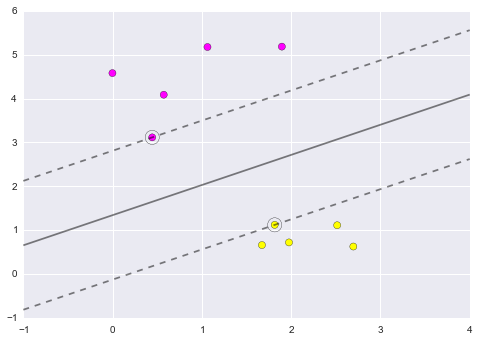Notice the unique thing about SVM is that only the support vectors matter: that is, if you moved any of the other points without letting them cross the decision boundaries, they would have no effect on the classification results!

#### Going further: Kernel Methods¶

Where SVM gets incredibly exciting is when it is used in conjunction with kernels. To motivate the need for kernels, let's look at some data which is not linearly separable:

In :
from sklearn.datasets.samples_generator import make_circles
X, y = make_circles(100, factor=.1, noise=.1)

clf = SVC(kernel='linear').fit(X, y)

plt.scatter(X[:, 0], X[:, 1], c=y, s=50, cmap='spring')
plot_svc_decision_function(clf);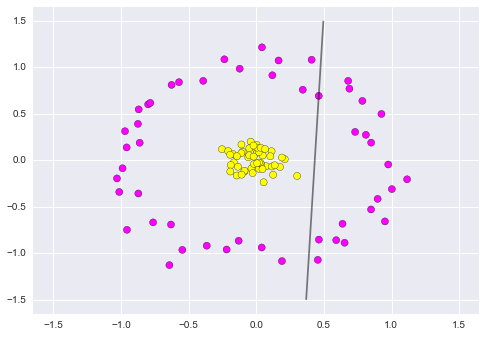Clearly, no linear discrimination will ever separate these data. One way we can adjust this is to apply a kernel, which is some functional transformation of the input data.

For example, one simple model we could use is a radial basis function

In :
r = np.exp(-(X[:, 0] ** 2 + X[:, 1] ** 2))


If we plot this along with our data, we can see the effect of it:

In :
from mpl_toolkits import mplot3d

def plot_3D(elev=30, azim=30):
ax = plt.subplot(projection='3d')
ax.scatter3D(X[:, 0], X[:, 1], r, c=y, s=50, cmap='spring')
ax.view_init(elev=elev, azim=azim)
ax.set_xlabel('x')
ax.set_ylabel('y')
ax.set_zlabel('r')

interact(plot_3D, elev=[-90, 90], azip=(-180, 180));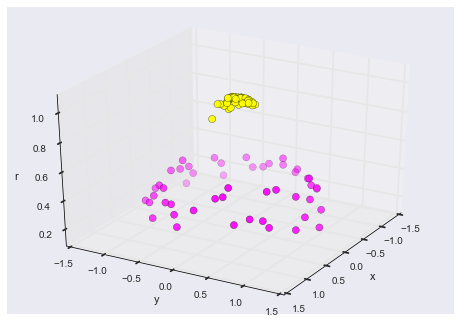We can see that with this additional dimension, the data becomes trivially linearly separable! This is a relatively simple kernel; SVM has a more sophisticated version of this kernel built-in to the process. This is accomplished by using kernel='rbf', short for radial basis function:

In :
clf = SVC(kernel='rbf')
clf.fit(X, y)

plt.scatter(X[:, 0], X[:, 1], c=y, s=50, cmap='spring')
plot_svc_decision_function(clf)
plt.scatter(clf.support_vectors_[:, 0], clf.support_vectors_[:, 1],
s=200, facecolors='none');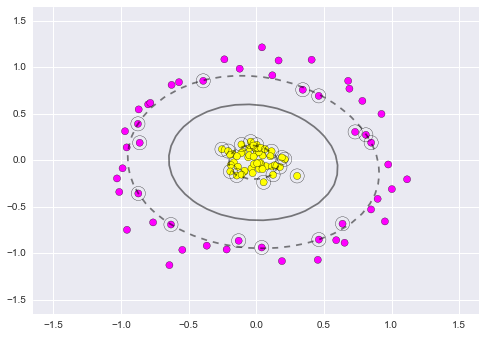Here there are effectively $N$ basis functions: one centered at each point! Through a clever mathematical trick, this computation proceeds very efficiently using the "Kernel Trick", without actually constructing the matrix of kernel evaluations.

We'll leave SVMs for the time being and take a look at another classification algorithm: Random Forests.